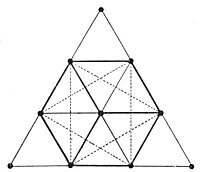## Posts

Showing posts from November, 2012

### Tεtrαctys ~ GEOMETRY ~ PYTHAGORAS/PYTHAGORUSAt 582 BC the Greek philosopher and mathematician Pythagoras, from which we got the Pythagorean Theorem in geometry, once called the tetractys the symbol of the musical, arithmetic and geometric ratios upon which the universe is built. For Pythagoras and his followers, each line of the tetractys holds these meanings: The Tetractys was considered a divine blueprint of creation and found many uses in Math, Medicine, Music, etc. The Tetractys contains four rows with a successive number of dots 1, 2, 3, and 4, hence the name Tetractys. The decimal numbers 0-9 can be assigned to each of the ten dots that form an equilateral triangle. In the center we find the lowest number zero, that represents unrealized potential. Zero was the source of all that came into being, represented by the highest number nine. Nine thus represents All-One-ness, At-One-ment. Since nine wanted to create other things it had to divide itself into two parts: six and three. Six represents form, memory, and space. T